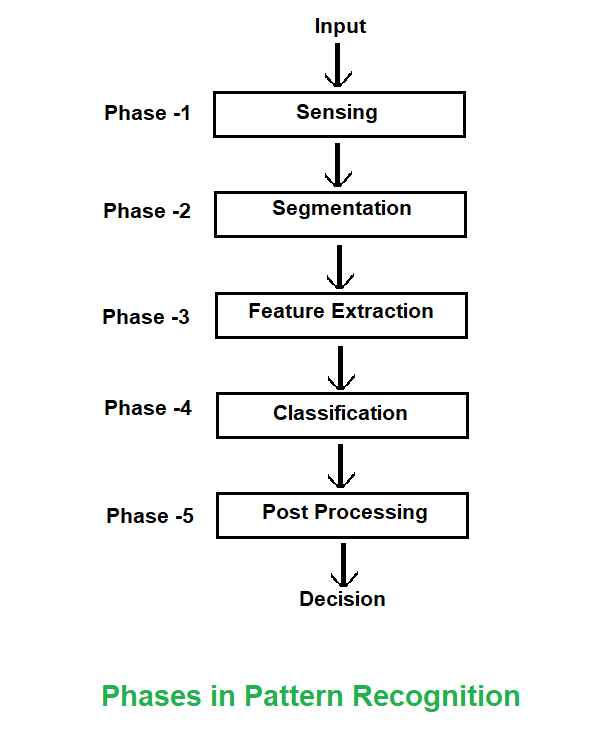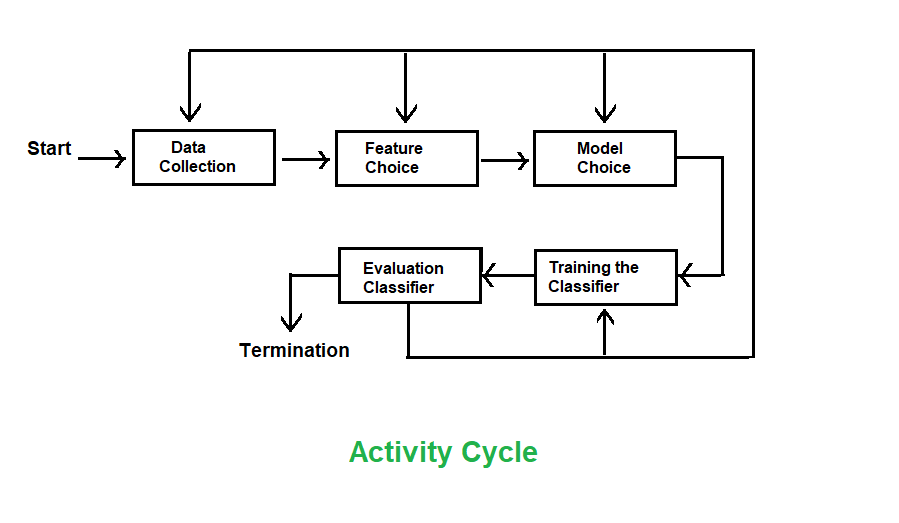Open in App
Not now

# Pattern Recognition | Phases and Activities

• Difficulty Level : Expert
• Last Updated : 10 Jan, 2023

Prerequisite – Pattern Recognition | Introduction
Phases in Pattern Recognition System
Approaches for Pattern Recognition Systems can be represented by distinct phases, as Pattern Recognition Systems can be divided into the following components.

• Phase 1: Convert images or sounds or other inputs into signal data.
• Phase 2: Isolate the sensed objects from the background.
• Phase 3: Measure objects properties that are useful for classification.
• Phase 4: Assign the sensed object to a category.
• Phase 5: Take other considerations to decide on appropriate action.Problems solved by these Phases are as follows:

1. Sensing: It deals with problem arises in the input such as its bandwidth, resolution, sensitivity, distortion, signal-to-noise ratio, latency, etc.
2. Segmentation and Grouping: Deepest problems in pattern recognition that deals with the problem of recognizing or grouping together the various parts of an object.
3. Feature Extraction: It deals with the characterization of an object so that it can be recognized easily by measurements. Those objects whose values are very similar for the objects are considered to be in the same category, while those whose values are quite different for the objects are placed in different categories.
4. Classification: It deals with assigning the object to their particular categories by using the feature vector provided by the feature extractor and determining the values of all of the features for a particular input.
5. Post Processing: It deals with action decision-making by using the output of the classifier. Action such as minimum-error-rate classification will minimize the total expected cost.

Activities for designing the Pattern Recognition Systems

There are various sequences of activities that are used for designing the Pattern Recognition Systems. These activities are as follows:

• Data Collection
• Feature Choice
• Model Choice
• Training
• EvaluationThere are typically four main phases in the pattern recognition process: preprocessing, training, testing, and deployment. These phases involve a series of activities that are designed to develop and evaluate a pattern recognition system.

Preprocessing: Preprocessing is the process of preparing the data for analysis. This may involve cleaning the data, scaling the data, or transforming the data in some way to make it more suitable for analysis.

Training: Training is the process of fitting a model to the data. This typically involves selecting a model, choosing appropriate hyperparameters, and optimizing the model’s parameters to minimize a loss function.

Testing: Testing is the process of evaluating the performance of the model on a held-out dataset. This allows us to estimate the generalization performance of the model and to compare the performance of different models.

Deployment: Deployment is the process of deploying the trained model in a production environment. This may involve integrating the model into an existing system or building a new system based on the model.

## Python3

 `import` `numpy as np` `# Utility function to compute the Euclidean distance between two points``def` `euclidean_distance(x, y):``    ``return` `np.sqrt(np.``sum``((x ``-` `y) ``*``*` `2``))` `# Utility function to find the k nearest neighbors of a point``def` `find_nearest_neighbors(point, points, k``=``5``):``    ``distances ``=` `[euclidean_distance(point, p) ``for` `p ``in` `points]``    ``nearest_neighbors ``=` `np.argsort(distances)[:k]``    ``return` `nearest_neighbors`

My Personal Notes arrow_drop_up